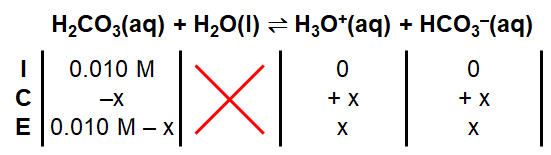# Problem: Calculate [CO32-] in a 0.010-M solution of CO2 in water (usually written as H2CO3). If all the CO32- in this solution comes from the reactionHCO3-(aq) ⇌ H+(aq) + CO32-(aq)what percentage of the H+ ions in the solution is a result of the dissociation of HCO3-? When acid is added to a solution of sodium hydrogen carbonate (NaHCO3), vigorous bubbling occurs. How is this reaction related to the existence of carbonic acid (H2CO3) molecules in aqueous solution?

###### FREE Expert Solution

Given: 0.010 M HCO3               Ka1 = 4.5 × 10–7               Ka2 = 4.7 × 10–11

Part A:

ICE Chart for Ka1:98% (169 ratings)###### Problem Details

Calculate [CO32-] in a 0.010-M solution of CO2 in water (usually written as H2CO3). If all the CO32- in this solution comes from the reaction

HCO3-(aq) ⇌ H+(aq) + CO32-(aq)

what percentage of the H+ ions in the solution is a result of the dissociation of HCO3-? When acid is added to a solution of sodium hydrogen carbonate (NaHCO3), vigorous bubbling occurs. How is this reaction related to the existence of carbonic acid (H2CO3) molecules in aqueous solution?

Frequently Asked Questions

What scientific concept do you need to know in order to solve this problem?

Our tutors have indicated that to solve this problem you will need to apply the Diprotic Acid concept. You can view video lessons to learn Diprotic Acid. Or if you need more Diprotic Acid practice, you can also practice Diprotic Acid practice problems.

What professor is this problem relevant for?

Based on our data, we think this problem is relevant for Professor Liwosz's class at UB.

What textbook is this problem found in?

Our data indicates that this problem or a close variation was asked in Chemistry: An Atoms First Approach - Zumdahl Atoms 1st 2nd Edition. You can also practice Chemistry: An Atoms First Approach - Zumdahl Atoms 1st 2nd Edition practice problems.